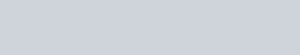# Symmetry of length and duration

There is a symmetry principle for length and duration:

Measures of length and duration are interchangeable and the forms of equations remain the same if all measures of length are interchanged with their corresponding measures of duration and vice versa. The three-dimensionality of length is fully reflected in the three-dimensionality of duration.

This is a physical principle that does not imply or depend on an invariant one-way speed of light. It is an artifact of the act of measurement, which may be developed in a strictly symmetric manner.

Einstein’s theory of relativity implies that in some way “space” and “time” are the same thing or different aspects of the same thing or may be exchanged. For example, Field articulates a Kinematical Special Relativity Principle (KSRP) as a consequence of the Lorentz transform:

‘‘The equations describing the laws of physics are invariant with respect to the exchange of space and time coordinates, or, more generally, to the exchange of the spatial and temporal components of four vectors.’’ [Space-time exchange invariance: Special relativity as a symmetry principle, J. H. Field: Citation: American Journal of Physics 69, 569 (2001); doi: 10.1119/1.1344165]

However, the three-dimensionality of “space” versus the one-dimensionality of “time” is an incomplete symmetry. A different approach is needed to overcome this.

Motion

The extent of every motion has two measures: its length and its duration. These are given as two quantities or one rate.

These quantities are measured by the alignment of two adjacent rigid structures or one rigid structure with an adjacent pointer. One of the rigid structures or the pointer is in uniform motion with respect to the other. If the observer is at rest relative to the structure at rest, then the measurement is a length. If the observer is at rest relative to the structure in uniform motion, then the measurement is a duration.